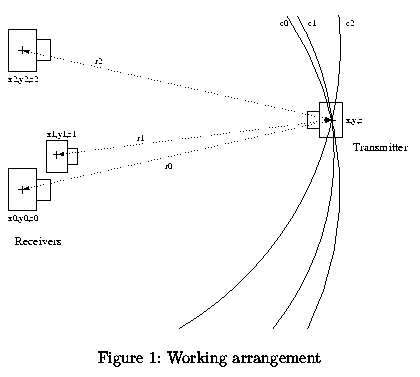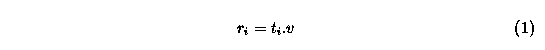### 2. How does it work

In articles ,  was published a simple method for position determination in 3D space with possible robotic application. These works gave an inspiration and basic knowledge about using ultrasonic waves for position sensing.

The idea of the 3D mouse is actually very simple. The basic elements of the 3D mouse are at least three ultrasonic receivers (non-collinear) and one transmitter as shown at fig. 1.The position in 3D space is calculated as follows :
1. Burst of the ultrasonic signal is emitted from the transmitter and "stop-watch" is started.
2. After arrival of the ultrasonic wave to the receiver, "stop-watch" is stopped and calculations start.
3. From the measured time and known velocity of the sound in the air (cca 340 m.s-1), distance to the each receiver is calculated. We use a simple formula (1), where ri is the calculated distance to the i-th receiver, ti is the measured time (TOF) and v is the velocity of the sound in the air.
4. Position in space is calculated from already known distances by solving equation system (2), where xi, yi, zi are known coordinates of receivers, ri is the calculated distance from transmitter to the corresponding receiver and finally x, y, z are coordinates determining position of the transmitter in 3D space. System (2) can actually have two solutions, but one of themcan be safely ignored, because it describes a point behind the receivers plane.